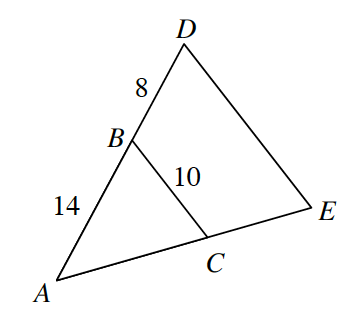### Home > CCG > Chapter 6 > Lesson 6.1.3 > Problem6-25

6-25.In the diagram at right, $ΔABC$ ~ $ΔADE$.

1. Draw each triangle separately on your paper. Be sure to include all measurements in your diagrams.

1. Find the length of $\overline{DE}$.

Set up a proportion to solve for $DE$.

$DE\approx15.71$